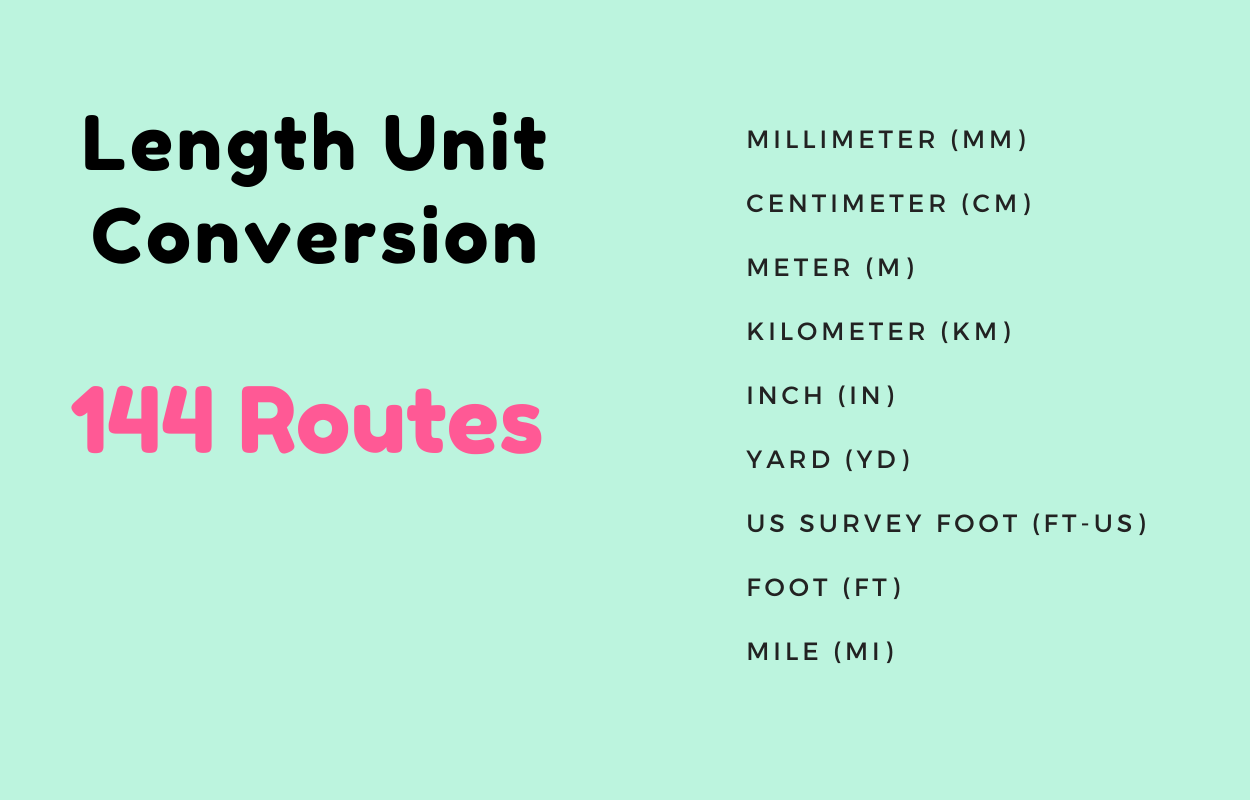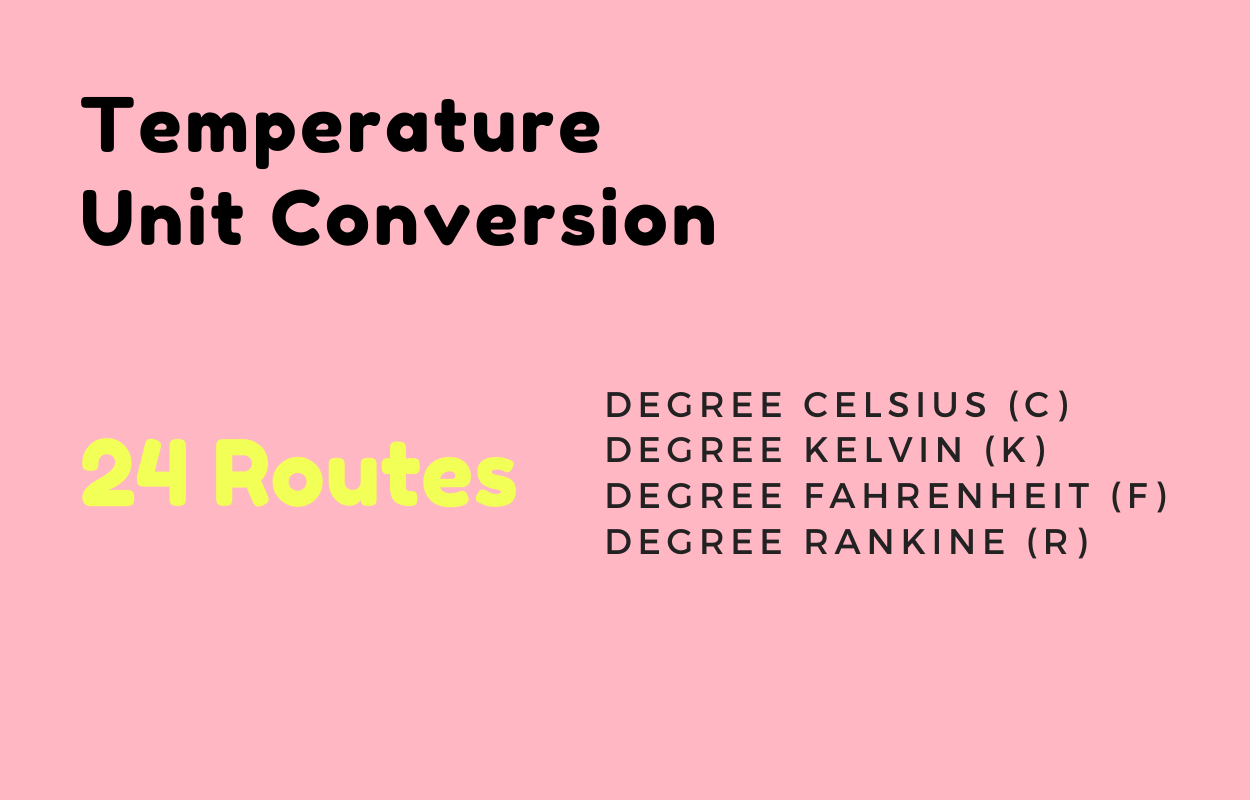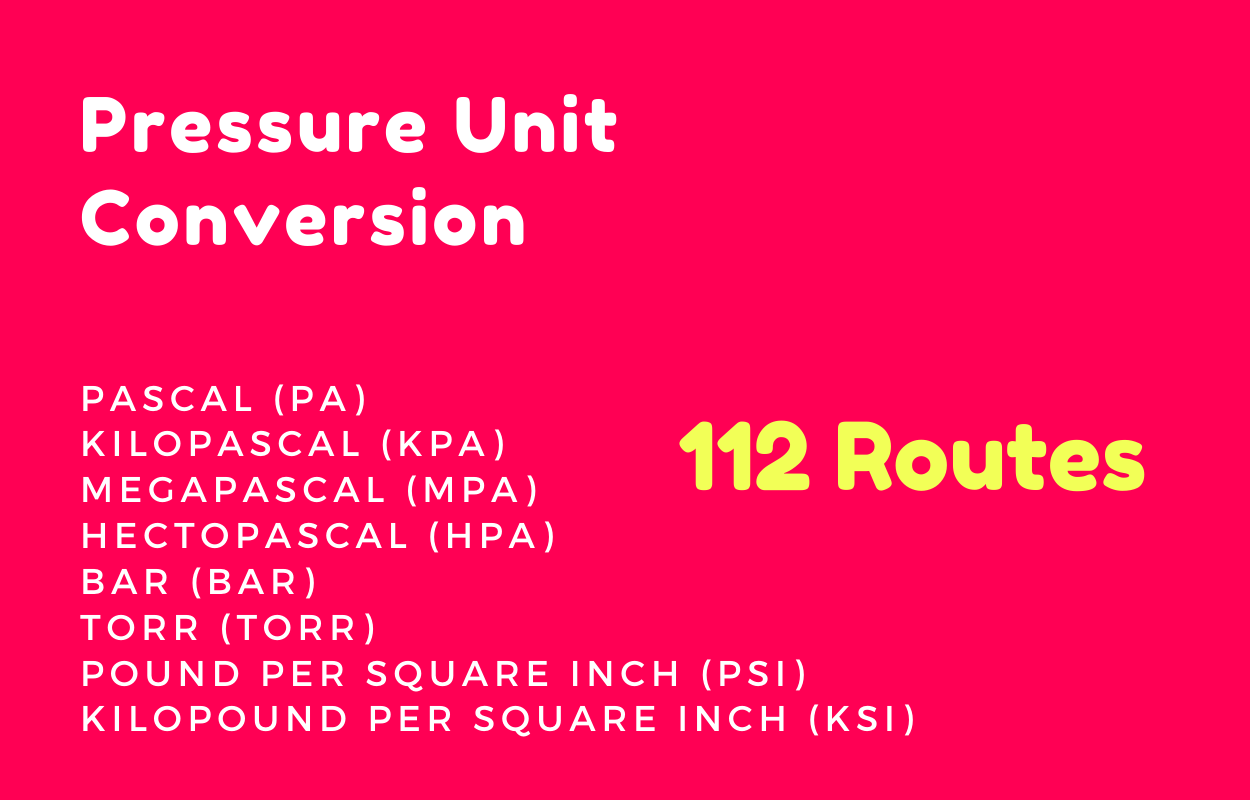GS

## Complete functional online unit converter tools developed by latest Angular 11## Website Features

• A handy utility for converting between quantities in different units
• There are a total of 23 supported units for converter and 173+ sub units for converter
• There are total 2883 routes in this application
• Every section of each conversion contains the main value of each unit
• Demo Website – https://appsplaces-unit-converter.firebaseapp.com/
• No backend and No external APIs
• NPM utility library used to measure and convert between units https://www.npmjs.com/package/convert-units
• Beautiful Designed
• Technologies: Angular 11, TypeScript
• Mobile Friendly
• Single Page Application
• Well Documentation and Code Comment
• Very Clean Code & Easily Customizable

## Supported Units

• mm
• cm
• m
• in
• ft-us
• ft
• fathom
• mi
• nMi

• mm2
• cm2
• m2
• ha
• km2
• in2
• ft2
• ac
• mi2

• mcg
• mg
• g
• kg
• oz
• lb
• mt
• t

• mm3
• cm3
• ml
• l
• kl
• m3
• km3
• tsp
• Tbs
• in3
• fl-oz
• cup
• pnt
• qt
• gal
• ft3
• yd3

• mm3/s
• cm3/s
• ml/s
• cl/s
• dl/s
• l/s
• l/min
• l/h
• kl/s
• kl/min
• kl/h
• m3/s
• m3/min
• m3/h
• km3/s
• tsp/s
• Tbs/s
• in3/s
• in3/min
• in3/h
• fl-oz/s
• fl-oz/min
• fl-oz/h
• cup/s
• pnt/s
• pnt/min
• pnt/h
• qt/s
• gal/s
• gal/min
• gal/h
• ft3/s
• ft3/min
• ft3/h
• yd3/s
• yd3/min
• yd3/h’

• C
• F
• K
• R

• ns
• mu
• ms
• s
• min
• h
• d
• week
• month
• year

• Hz
• mHz
• kHz
• MHz
• GHz
• THz
• rpm
• deg/s

• m/s
• km/h
• m/h
• knot
• ft/s

• s/m
• min/km
• s/ft
• min/km

• Pa
• hPa
• kPa
• MPa
• bar
• torr
• psi
• ksi

• b
• Kb
• Mb
• Gb
• Tb
• B
• KB
• MB
• GB
• TB

• lx
• ft-cd

• ppm
• ppb
• ppt
• ppq

• V
• mV
• kV

• A
• mA
• kA

• W
• mW
• kW
• MW
• GW

• VA
• mVA
• kVA
• MVA
• GVA

• VAR
• mVAR
• kVAR
• MVAR
• GVAR

• Wh
• mWh
• kWh
• MWh
• GWh
• J
• kJ

• VARh
• mVARh
• kVARh
• MVARh
• GVARh

• deg
• arcmin
• arcsec

• c
• mC
• μC
• nC
• pC

• N
• kN
• lbf

### Acceleration

• g (g-force)
• m/s2